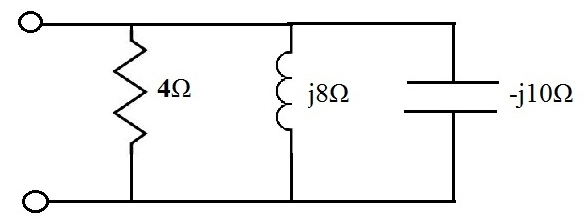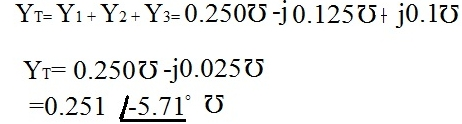﻿ What is the Admittance of a Circuit?# What is the Admittance of a Circuit?

The Admittance of a circuit is the measure of the circuit's conductance, which is its ability to conduct electric current through it. Being that admittance is the measure of a circuit's conductance, it is the direct opposite of the circuit's impedance, its opposition to the flow of current; so, thus, to calculate admittance, the formula (1/Impedance) is used. Admittance is measured in a unit called siemens ().

Let's now take a look at a circuit, as an example, and calculate its admittance so you can see a real-world problem of it:Since all the impedance values of the components, the resistor, capacitor, and inductor, are given, all we have to do is use the formula, 1/impedance, to calculate each of their admittance values. Below are the calculations:Now that we have all the admittance values of the components, all we must do now is simply add them to get the final answer. Here we end up putting the final answer in polar form shown below.Related Resources

HTML Comment Box is loading comments...